# Spectrum Sensing with Deep Learning to Identify 5G and LTE Signals

This example shows how to train a semantic segmentation network using deep learning for spectrum monitoring. One of the uses of spectrum monitoring is to characterize spectrum occupancy. The neural network in this example is trained to identify 5G NR and LTE signals in a wideband spectrogram.

### Introduction

Computer vision uses the semantic segmentation technique to identify objects and their locations in an image or a video. In wireless signal processing, the objects of interest are wireless signals, and the locations of the objects are the frequency and time occupied by the signals. In this example we apply the semantic segmentation technique to wireless signals to identify spectral content in a wideband spectrogram.

In the following, you will:

1. Generate training signals.

2. Use a semantic segmentation network to identify 5G NR and LTE signals in time and frequency. You have the choice of training a network from scratch or applying transfer learning to a pretrained network.

3. Test the trained network with synthetic signals.

4. Use an SDR to test the network with over the air (OTA) signals.

#### Associated AI for Wireless Examples

Use this example as part of a complete deep learning workflow:

1. The Capture and Label NR and LTE Signals for AI Training (Wireless Testbench) example shows how to scan, capture, and label bandwidths with 5G NR and LTE signals using a SDR.

2. This example shows how to train a semantic segmentation network to identify 5G NR and LTE signals in a wideband spectrogram.

3. The Identify LTE and NR Signals from Captured Data Using SDR and Deep Learning (Wireless Testbench) example shows how to use a deep learning trained semantic segmentation network to identify NR and LTE signals from wireless data captured with a SDR.

The intended workflow of the examples above is shown in the diagram.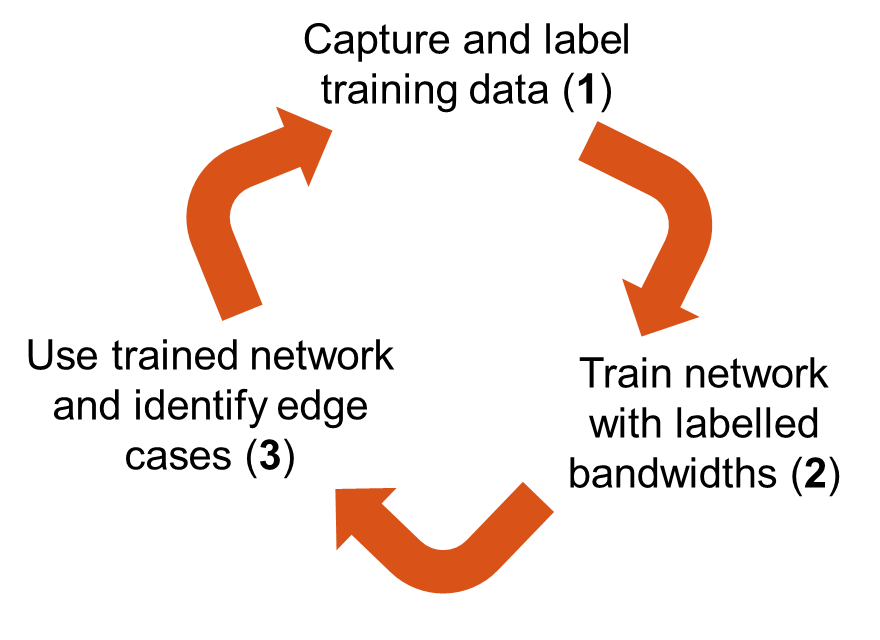### Generate Training Data

One advantage of wireless signals in the deep learning domain is the fact that the signals are synthesized. Also, we have highly reliable channel and RF impairment models. As a result, instead of collecting and manually labeling signals, you can generate 5G NR signals using 5G Toolbox™ and LTE signals using LTE Toolbox™ functions. You can pass these signals through standards-specified channel models to create the training data.

Train the network with frames that contain only 5G NR or LTE signals and then shift these signals in frequency randomly within the band of interest. Each frame is 40 ms long, which is the duration of 40 subframes. The network assumes that the 5G NR or LTE signal occupies the same band for the whole frame duration. To test the network performance, create frames that contain both 5G NR and LTE signals on distinct random bands within the band of interest.

Use a sampling rate of 61.44 MHz. This rate is high enough to process most of the latest standard signals and several low-cost software defined radio (SDR) systems can sample at this rate providing about 50 MHz of useful bandwidth. To monitor a wider band, you can increase the sample rate, regenerate training frames and retrain the network.

Use the `helperSpecSenseTrainingData` function to generate training frames. This function generates 5G NR signals using the `helperSpecSenseNRSignal` function and LTE signals using the `helperSpecSenseLTESignal` function. This table lists 5G NR variable signal parameters.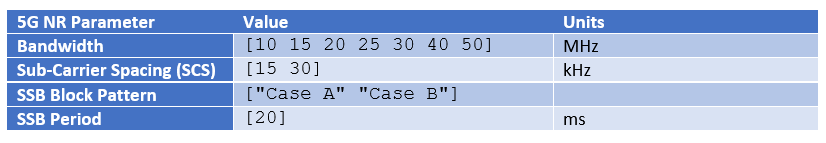This table lists LTE variable signal parameters.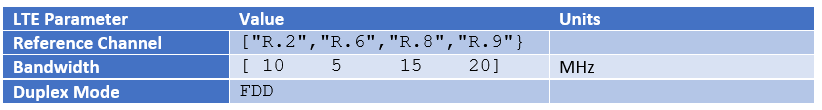Use the `nrCDLChannel` (5G Toolbox) and the `lteFadingChannel` (LTE Toolbox) functions to add channel impairments. For details of the channel configurations, see the `helperSpecSenseTrainingData` function. This table lists channel parameters.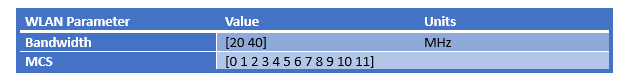The `helperSpecSenseTrainingData` function uses the `helperSpecSenseSpectrogramImage` function to create spectrogram images from complex baseband signals. Calculate the spectrograms using an FFT length of 4096. Generate 128 by 128 RGB images. This image size allows a large enough batch of images to fit in memory during training while providing enough resolution in time and frequency. If your GPU does not have sufficient memory, you can resize the images to smaller sizes or reduce the training batch size.

The `generateTrainData` variable determines whether training data is to be downloaded or generated. Choosing "Use downloaded data" sets the `generateTrainData` variable to `false`. Choosing "Generate training data" sets the `generateTrainData` variable to `true` to generate the training data from scratch. Data generation may take several hours depending on the configuration of your computer. Using a PC with Intel® Xeon® W-2133 CPU @ 3.60GHz and creating a parallel pool with six workers with the Parallel Computing Toolbox™, training data generation takes about an hour. Choose "Train network now" to train the network. This process takes about 8 minutes with the same PC and NVIDIA® Titan V GPU. Choose "Use trained network" to skip network training. Instead, the example downloads the trained network.

Use 900 frames from each set of signals: 5G NR only, LTE only and 5G NR and LTE both. If you increase the number of possible values for the system parameters, increase the number of training frames.

Set `imageSize` to a cell array of image sizes. You generate a set of training images for each specified size. In this example, you use 128-by-128 RGB images to train a semantic segmentation network from scratch. You use 256-by-256 images to apply transfer learning to pretrained semantic segmentation networks.

The downloaded training data also includes captured, preprocessed, and labeled data for LTE, 5G, and unknown signals over a 250 MHz band. See the Capture and Label NR and LTE Signals for AI Training (Wireless Testbench) example for more details.

```imageSize = {[256 256], [128 128]}; % pixels sampleRate = 61.44e6; % Hz numSubFrames = 40; % corresponds to 40 ms frameDuration = numSubFrames*1e-3; % seconds trainDir = fullfile(pwd,"TrainingData"); classNames = ["Noise" "NR" "LTE" "Unknown"]; generateTrainData =false; trainNow =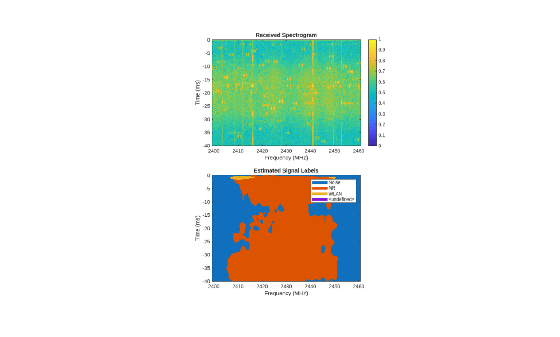false; if generateTrainData numFramesPerStandard = 900; saveChannelInfo = false; helperSpecSenseTrainingData(numFramesPerStandard,classNames,imageSize,trainDir,numSubFrames,sampleRate,saveChannelInfo); end```

### Choose Deep Neural Network

You have the choice of training a semantic segmentation network from scratch, or applying transfer learning to a pretrained semantic segmentation network.

• To train a custom network from scratch, set `baseNetwork` to "custom". The custom network trains on 128-by-128 RGB images.

• To apply transfer learning, set `baseNetwork` to the desired pretrained network architecture.The pretrained networks train on 256-by-256 RGB images.

```baseNetwork =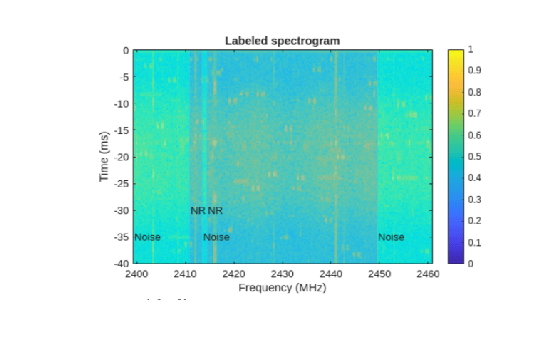'custom'; if strcmp(baseNetwork,"custom") trainDir = fullfile(trainDir,'128x128'); imageSize = [128 128]; else trainDir = fullfile(trainDir,'256x256'); %#ok imageSize = [256 256]; end```

```if ~generateTrainData || ~trainNow helperSpecSenseDownloadData(generateTrainData,trainNow,baseNetwork,imageSize) end```
```Starting download of data files from: https://www.mathworks.com/supportfiles/spc/SpectrumSensing/SpectrumSensingTrainingData128x128.tgz Extracting files. Extract complete. Starting download of data files from: https://www.mathworks.com/supportfiles/spc/SpectrumSensing/SpectrumSensingTrainedCustom.zip Extracting files. Extract complete. ```

Use the `imageDatastore` function to load training images with the spectrogram of 5G NR and LTE signals. The `imageDatastore` function enables you to efficiently load a large collection of images from disk. Spectrogram images are stored in .png files.

```folders = [trainDir,fullfile(trainDir,"captured")]; imds = imageDatastore(folders,FileExtensions=".png");```

Use the `pixelLabelDatastore` (Computer Vision Toolbox) function to load spectrogram pixel label image data. Each pixel is labeled as one of "NR", "LTE", "Noise", or "Unknown". A pixel label datastore encapsulates the pixel label data and the label ID to a class name mapping. Pixel labels are stored in .hdf files.

```numClasses = length(classNames); pixelLabelID = floor((0:numClasses-1)/(numClasses-1)*255); pxdsTruthLTENR = pixelLabelDatastore(folders,classNames,pixelLabelID,... FileExtensions=".hdf");```

#### Analyze Dataset Statistics

To see the distribution of class labels in the training dataset, use the `countEachLabel` (Computer Vision Toolbox) function to count the number of pixels by class label, and plot the pixel counts by class.

```tbl = countEachLabel(pxdsTruthLTENR); frequency = tbl.PixelCount/sum(tbl.PixelCount); figure bar(1:numel(classNames),frequency) grid on xticks(1:numel(classNames)) xticklabels(tbl.Name) xtickangle(45) ylabel("Frequency")```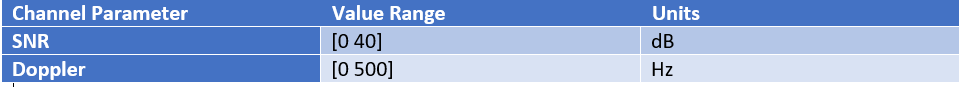Ideally, all classes would have an equal number of observations. However, with wireless signals it is common for the classes in the training set to be imbalanced. 5G NR signals may have larger bandwidth than LTE signals, and noise fills the background. Because the learning is biased in favor of the dominant classes, imbalance in the number of observations per class can be detrimental to the learning process. In the Balance Classes Using Class Weighting section, class weighting is used to mitigate bias caused by imbalance in the number of observations per class.

#### Prepare Training, Validation, and Test Sets

The deep neural network uses 80% of the signal images from the dataset for training, 10% of the images for validation, and 10% of the images for testing. The `helperSpecSensePartitionData` function randomly splits the image and pixel label data into training,validation, and test sets.

```[imdsTrain,pxdsTrain,imdsVal,pxdsVal,imdsTest,pxdsTest] = ... helperSpecSensePartitionData(imds,pxdsTruthLTENR,[80 10 10]); cdsTrain = combine(imdsTrain,pxdsTrain); cdsVal = combine(imdsVal,pxdsVal); cdsTest = combine(imdsTest,pxdsTest);```

Apply a transform to resize the image and pixel label data to the desired size.

```cdsTrain = transform(cdsTrain, @(data)preprocessTrainingData(data,imageSize)); cdsVal = transform(cdsVal, @(data)preprocessTrainingData(data,imageSize)); cdsTest = transform(cdsTest, @(data)preprocessTrainingData(data,imageSize));```

### Train Deep Neural Network

You have the choice of training a network from scratch or applying transfer learning.

#### Design a Simple Semantic Segmentation Network

In this section, you design a simple semantic segmentation network.If you want to use transfer learning on a pretrained network instead, you can skip this section and proceed to Use a Pretrained Network for Transfer Learning.

A common pattern in semantic segmentation networks requires the downsampling of an image between convolutional and ReLU layers, and then upsampling the output to match the input size. During this process, a network performs the operations using non-linear filters optimized for a specific set of classes that you want to segment.

First, define the input image layer.

```inputSize = [imageSize(1) imageSize(2) 3]; imgLayer = imageInputLayer(inputSize);```

Next, define the downsampling network, comprised of convolution and ReLU layers. The convolution layer padding is selected such that the output size of the convolution layer is the same as the input size. This makes it easier to construct a network because the input and output sizes between most layers remain the same as you progress through the network.

The downsampling is performed using a max pooling layer. Create a max pooling layer to downsample the input by a factor of 2 by setting the '`Stride`' parameter to 2.

```filterSize = 3; numFilters = 128; conv = convolution2dLayer(filterSize,numFilters,'Padding',1); relu = reluLayer(); poolSize = 2; maxPoolDownsample2x = maxPooling2dLayer(poolSize,'Stride',2);```

Stack the convolution, ReLU, and max pooling layers to create a network that downsamples its input by a factor of 16.

```downsamplingLayers = [ conv relu maxPoolDownsample2x conv relu maxPoolDownsample2x conv relu maxPoolDownsample2x conv relu maxPoolDownsample2x ];```

Next, create the upsampling network. The upsampling is done using the transposed convolution layer (also commonly referred to as "deconv" or "deconvolution" layer). When a transposed convolution is used for upsampling, it performs the upsampling and the filtering at the same time.

Create a transposed convolution layer to upsample by 2.

```filterSize = 4; transposedConvUpsample2x = transposedConv2dLayer(filterSize,numFilters,'Stride',2,'Cropping',1);```

The 'Cropping' parameter is set to 1 to make the output size equal twice the input size.

Stack the transposed convolution and relu layers. An input to this set of layers is upsampled by 16.

```upsamplingLayers = [ transposedConvUpsample2x relu transposedConvUpsample2x relu transposedConvUpsample2x relu transposedConvUpsample2x relu ];```

The final set of layers are responsible for making pixel classifications. These final layers process an input that has the same spatial dimensions (height and width) as the input image. However, the number of channels (third dimension) is larger and is equal to number of filters in the last transposed convolution layer. This third dimension needs to be squeezed down to the number of classes we wish to segment. This can be done using a 1-by-1 convolution layer whose number of filters equal the number of classes (here, 3).

Create a convolution layer to combine the third dimension of the input feature maps down to the number of classes.

`conv1x1 = convolution2dLayer(1,numClasses);`

Following this 1-by-1 convolution layer are the softmax and classification layers. These two layers combine to predict the categorical label for each image pixel.

```finalLayers = [ conv1x1 softmaxLayer() classificationLayer("Name","classification") ];```

Stack all the layers to complete the semantic segmentation network.

```layers = [ imgLayer downsamplingLayers upsamplingLayers finalLayers ]; lgraph = layerGraph(layers);```

#### Use a Pretrained Network for Transfer Learning

Instead of training a network from scratch, you can apply transfer learning to a popular semantic segmentation network.

To apply transfer learning, use the `deeplabv3plusLayers` (Computer Vision Toolbox) function to create a semantic segmentation neural network. Choose `resnet50` (Deep Learning Toolbox) as the base network (by setting the value of `baseNetwork`) and specify the input image size (number of pixels used to represent time and frequency axes) and the number of classes. If the Deep Learning Toolbox™ Model for ResNet-50 Network support package is not installed, then the function provides a link to the required support package in the Add-On Explorer. To install the support package, click the link, and then click Install. Check that the installation is successful by typing `resnet50` at the command line. If the required support package is installed, then the function returns a `DAGNetwork` (Deep Learning Toolbox) object.

```if ~strcmp(baseNetwork,"custom") lgraph = deeplabv3plusLayers([256 256],numel(classNames),baseNetwork);%#ok end```

#### Balance Classes Using Class Weighting

To improve training when classes in the training set are not balanced, you can use class weighting to balance the classes. Use the pixel label counts computed earlier with the `countEachLabel` function and calculate the median frequency class weights.

```imageFreq = tbl.PixelCount ./ tbl.ImagePixelCount; classWeights = median(imageFreq) ./ imageFreq;```

Specify the class weights using a `pixelClassificationLayer` (Computer Vision Toolbox).

```pxLayer = pixelClassificationLayer(Name="labels",Classes=tbl.Name,ClassWeights=classWeights); lgraph = replaceLayer(lgraph,"classification",pxLayer);```

#### Select Training Options

Configure training using the `trainingOptions` (Deep Learning Toolbox) function to specify the stochastic gradient descent with momentum (SGDM) optimization algorithm and the hyper-parameters used for SGDM. To get the best performance from the network, you can use the Experiment Manager (Deep Learning Toolbox) to optimize training options.

```mbs = 40; opts = trainingOptions("sgdm",... MiniBatchSize = mbs,... MaxEpochs = 20, ... LearnRateSchedule = "piecewise",... InitialLearnRate = 0.02,... LearnRateDropPeriod = 10,... LearnRateDropFactor = 0.1,... ValidationData = cdsVal,... ValidationPatience = 5,... Shuffle="every-epoch",... OutputNetwork = "best-validation-loss",... Plots = 'training-progress');```

Train the network using the combined training data store, `cdsTrain`. The combined training data store contains single signal frames and true pixel labels.

```if trainNow [net,trainInfo] = trainNetwork(cdsTrain,lgraph,opts); save(sprintf('myNet_%s_%s',baseNetwork, ... datetime('now',format='yyyy_MM_dd_HH_mm')), 'net') else net = loadNetworkFromMATFile(baseNetwork); %#ok end```

### Test Deep Neural Network

Test the network signal identification performance using signals that contain both 5G NR and LTE signals. Use the `semanticseg` (Computer Vision Toolbox) function to get the pixel estimates of the spectrogram images in the test data set. Use the `evaluateSemanticSegmentation` (Computer Vision Toolbox) function to compute various metrics to evaluate the quality of the semantic segmentation results.

```dataDir = fullfile(trainDir,"LTE_NR"); imdsLTENR = imageDatastore(dataDir,FileExtensions=".png"); pxdsResultsLTENR = semanticseg(imdsLTENR,net,MinibatchSize=mbs,WriteLocation=tempdir);```
```Running semantic segmentation network ------------------------------------- * Processed 897 images. ```
```pxdsTruthLTENR = pixelLabelDatastore(dataDir,classNames,pixelLabelID,... FileExtensions=".hdf"); metrics = evaluateSemanticSegmentation(pxdsResultsLTENR,pxdsTruthLTENR);```
```Evaluating semantic segmentation results ---------------------------------------- * Selected metrics: global accuracy, class accuracy, IoU, weighted IoU, BF score. * Processed 897 images. * Finalizing... Done. * Data set metrics: GlobalAccuracy MeanAccuracy MeanIoU WeightedIoU MeanBFScore ______________ ____________ _______ ___________ ___________ 0.91802 NaN 0.63619 0.84845 0.73257 ```

Plot the normalized confusion matrix for all test frames.

```cm = confusionchart(metrics.ConfusionMatrix.Variables, ... classNames, Normalization='row-normalized'); cm.Title = 'Confusion Matrix - Synthetic';```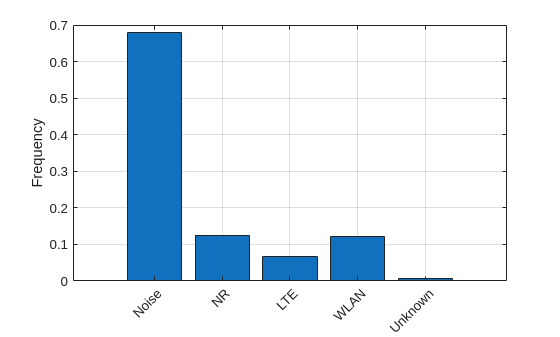Separate the test data into captured and generated sets.

```capturedIdx = contains(imdsTest.Files,'captured'); imdsTestCaptured = subset(imdsTest,capturedIdx); pxdsTestCaptured = subset(pxdsTest,capturedIdx); imdsTestGenerated = subset(imdsTest,~capturedIdx); pxdsTestGenerated = subset(pxdsTest,~capturedIdx);```

Repeat the same process, considering only the frames with captured data in the test set.

`pxdsResultsCaptured = semanticseg(imdsTestCaptured,net,MinibatchSize=mbs,WriteLocation=tempdir);`
```Running semantic segmentation network ------------------------------------- * Processed 54 images. ```
`metrics = evaluateSemanticSegmentation(pxdsResultsCaptured,pxdsTestCaptured);`
```Evaluating semantic segmentation results ---------------------------------------- * Selected metrics: global accuracy, class accuracy, IoU, weighted IoU, BF score. * Processed 54 images. * Finalizing... Done. * Data set metrics: GlobalAccuracy MeanAccuracy MeanIoU WeightedIoU MeanBFScore ______________ ____________ _______ ___________ ___________ 0.97702 0.96717 0.90162 0.95796 0.88535 ```

Replot the normalized confusion matrix.

```cm = confusionchart(metrics.ConfusionMatrix.Variables, ... classNames, Normalization="row-normalized"); cm.Title = "Normalized Confusion Matrix";```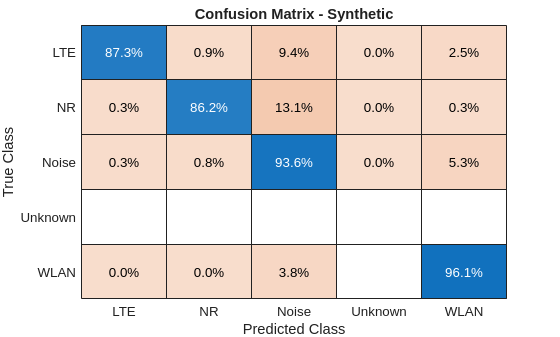The confusion matrix shows that the network confuses NR signals with Noise or Unknown signals. Examining the captured signals reveals that the captured signals with file prefix CF3550 has very low SNR and the network is having a hard time to identify signals correctly.

```CF3550Indices = contains(imdsTestCaptured.Files,'CF3550'); idx = find(CF3550Indices,1); rcvdSpectrogram = readimage(imdsTestCaptured,idx); trueLabels = readimage(pxdsTestCaptured,idx); predictedLabels = readimage(pxdsResultsCaptured,idx); figure helperSpecSenseDisplayResults(rcvdSpectrogram,trueLabels,predictedLabels, ... classNames,250e6,0,frameDuration)```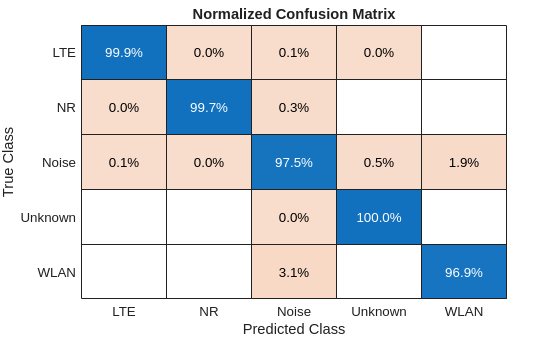Test with captured data but exclude CF3550 frames.

```imdsTestCaptured2 = subset(imdsTestCaptured,~CF3550Indices); pxdsTestCaptured2 = subset(pxdsTestCaptured,~CF3550Indices); pxdsResultsCaptured2 = semanticseg(imdsTestCaptured2,net,MinibatchSize=mbs,WriteLocation=tempdir);```
```Running semantic segmentation network ------------------------------------- * Processed 41 images. ```
`metrics = evaluateSemanticSegmentation(pxdsResultsCaptured2,pxdsTestCaptured2);`
```Evaluating semantic segmentation results ---------------------------------------- * Selected metrics: global accuracy, class accuracy, IoU, weighted IoU, BF score. * Processed 41 images. * Finalizing... Done. * Data set metrics: GlobalAccuracy MeanAccuracy MeanIoU WeightedIoU MeanBFScore ______________ ____________ _______ ___________ ___________ 0.99924 0.9983 0.9956 0.99849 0.99792 ```

Replot the normalized confusion matrix.

```figure cm = confusionchart(metrics.ConfusionMatrix.Variables, ... classNames, Normalization="row-normalized"); cm.Title = "Normalized Confusion Matrix";```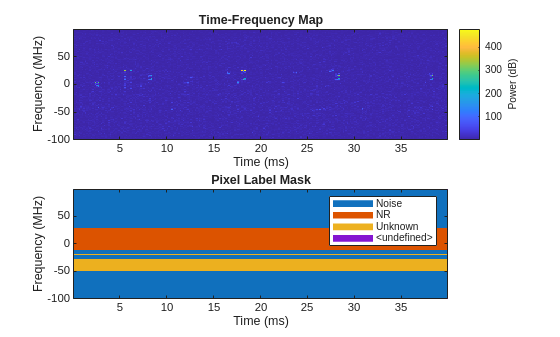The NR detection rate increases to more than 99%.

#### Identify 5G NR and LTE Signals in Spectrogram

Visualize the received spectrum, true labels, and predicted labels for a captured signal.

```signals = find(~CF3550Indices); numSignals = length(signals); idx =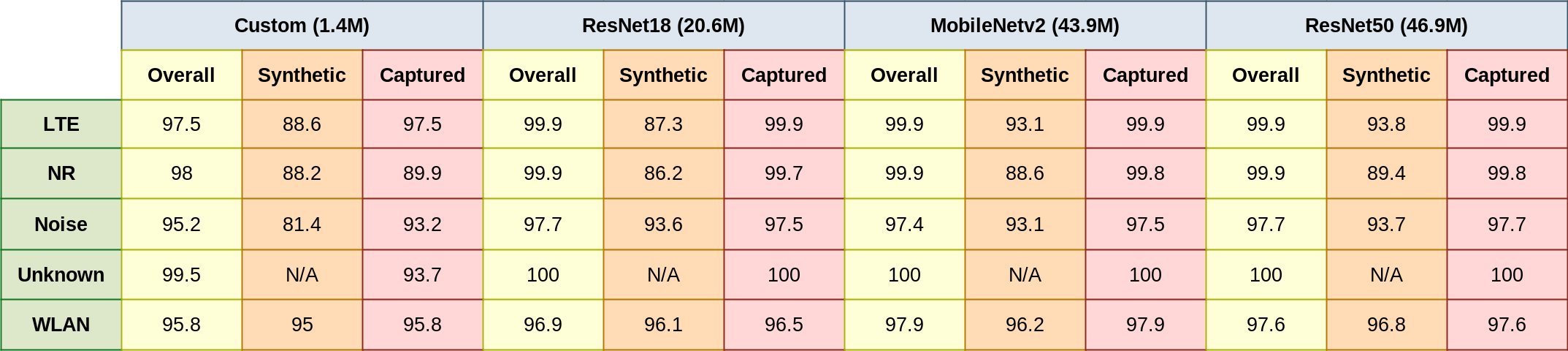13; rcvdSpectrogram = readimage(imdsTestCaptured,signals(idx)); trueLabels = readimage(pxdsTestCaptured,signals(idx)); predictedLabels = readimage(pxdsResultsCaptured,signals(idx)); figure helperSpecSenseDisplayResults(rcvdSpectrogram,trueLabels,predictedLabels, ... classNames,250e6,0,frameDuration)```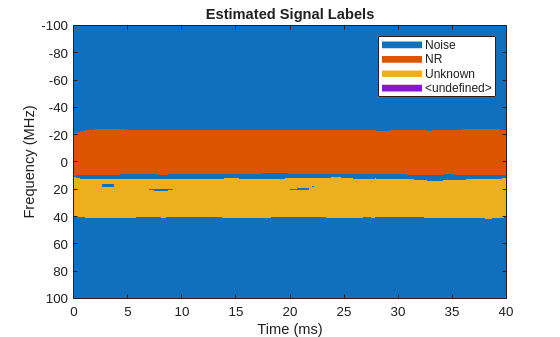```figure helperSpecSenseDisplayIdentifiedSignals(rcvdSpectrogram,predictedLabels, ... classNames,250e6,0,frameDuration)```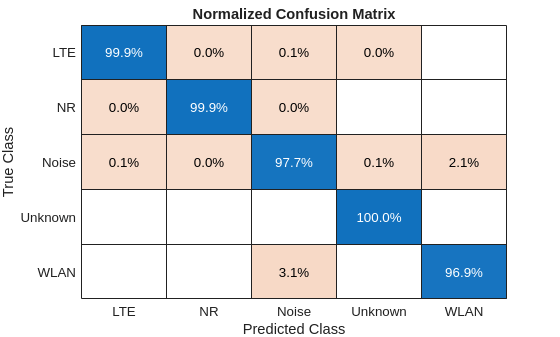### Test with Captured Data using SDR

Test the performance of the trained network using over-the-air signal captures using SDR. Find a nearby base station and tune the center frequency of your radio to cover the band of the signals you want to identify. This example sets the center frequency to 2.35 GHz. If you have at least one ADALM-PLUTO radio and have installed Communication Toolbox Support Package for ADALM-PLUTO Radio, you can run this section of the code. In case you do not have access to an ADALM-PLUTO radio, this example shows results of a test conducted using captured signals and a trained network.

Use Wireless Testbench example Identify LTE and NR Signals from Captured Data Using SDR and Deep Learning (Wireless Testbench) to test with wideband signals.

```runSDRSection = false; if helperIsPlutoSDRInstalled() radios = findPlutoRadio(); if length(radios) >= 1 runSDRSection = true; else disp("At least one ADALM-PLUTO radios is needed. Skipping SDR test.") end else disp("Communications Toolbox Support Package for Analog Devices ADALM-PLUTO Radio not found.") disp("Click Add-Ons in the Home tab of the MATLAB toolstrip to install the support package.") disp("Skipping SDR test.") end```
```Communications Toolbox Support Package for Analog Devices ADALM-PLUTO Radio not found. ```
```Click Add-Ons in the Home tab of the MATLAB toolstrip to install the support package. ```
```Skipping SDR test. ```
```if runSDRSection % Set up PlutoSDR receiver rx = sdrrx('Pluto'); rx.CenterFrequency = 2.35e9; rx.BasebandSampleRate = sampleRate; rx.SamplesPerFrame = frameDuration*rx.BasebandSampleRate; rx.OutputDataType = 'single'; rx.EnableBurstMode = true; rx.NumFramesInBurst = 1; Nfft = 4096; overlap = 10; meanAllScores = zeros([imageSize numel(classNames)]); segResults = zeros([imageSize 10]); for frameCnt=1:10 rxWave = rx(); rxSpectrogram = helperSpecSenseSpectrogramImage(rxWave,Nfft,sampleRate,imageSize); [segResults(:,:,frameCnt),scores,allScores] = semanticseg(rxSpectrogram,net); meanAllScores = (meanAllScores*(frameCnt-1) + allScores) / frameCnt; end release(rx) [~,predictedLabels] = max(meanAllScores,[],3); figure helperSpecSenseDisplayResults(rxSpectrogram,[],predictedLabels,classNames,... sampleRate,rx.CenterFrequency,frameDuration) figure freqBand = helperSpecSenseDisplayIdentifiedSignals(rxSpectrogram,predictedLabels,... classNames,sampleRate,rx.CenterFrequency,frameDuration) else figure imshow('lte_capture_result1.png') figure imshow('lte_capture_result2.png') figure imshow('nr_capture_result1.png') figure imshow('nr_capture_result2.png') end```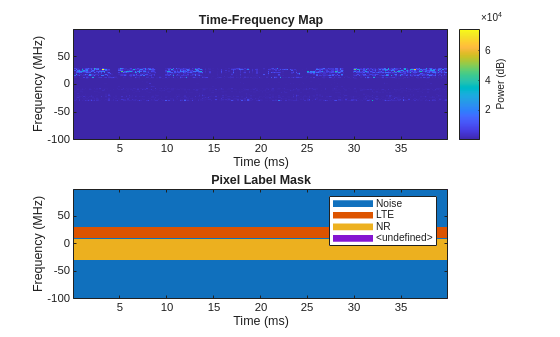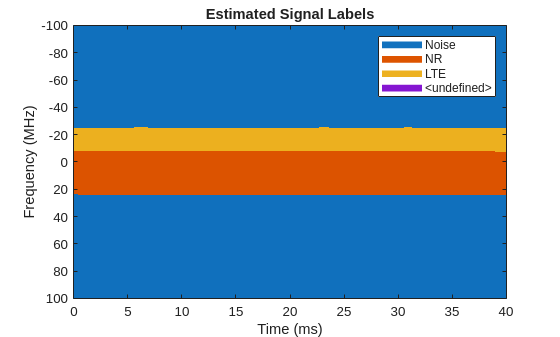### Conclusions and Further Exploration

The trained network can distinguish 5G NR and LTE signals including two example captures from real base stations. The network may not be able to identify every captured signal correctly. In such cases, enhance the training data either by generating more representative synthetic signals or capturing over-the-air signals and including these in the training set.

Different network structures result in different accuracy for detection. The table shows detection accuracy results for custom, ResNet-18, MobileNetv2, and ResNet-50, which have 1.4M, 20.6M, 43.9M and 46.9M learnables, respectively. Detection accuracy results are for overall test set, only synthetic signals, and only for captured signals. The overall and captured only tests include signals from the test set. The synthetic tests include generated signals in the LTE_NR directory, which are not used in training. Increasing the network complexity results in increased accuracy. But, with less than 1/20th learnables, the custom network looses only about 10% detection accuracy for captured NR signals as compared to MobileNetv2.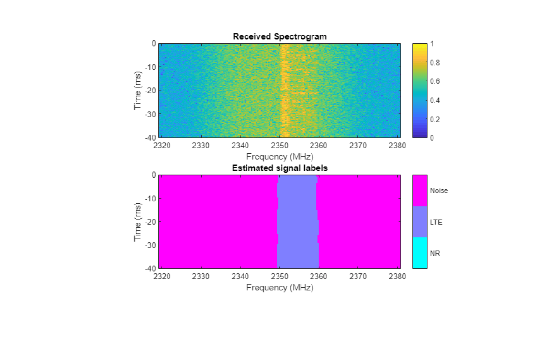You can use the Identify LTE and NR Signals from Captured Data Using SDR and Deep Learning (Wireless Testbench) example to identify LTE and 5G NR signals using the trained networks.

If you need to monitor wider bands of spectrum, increase the `sampleRate`, regenerate the training data, capture signals using the Capture and Label NR and LTE Signals for AI Training (Wireless Testbench) example, and retrain the network.

### Supporting Functions

```function data = preprocessTrainingData(data, imageSize) % Resize the training image and associated pixel label image. data{1} = imresize(data{1},imageSize); data{2} = imresize(data{2},imageSize); end function net = loadNetworkFromMATFile(baselineNetwork) switch baselineNetwork case "custom" net = load("specSenseTrainedNetCustom.mat",'net'); case "resnet18" net = load("specSenseTrainedNetResnet18.mat",'net'); case "resnet50" net = load("specSenseTrainedNetResnet50.mat",'net'); case "mobilenetv2" net = load("specSenseTrainedNetMobileNetv2.mat",'net'); otherwise error("Unknown baseline network: " + baselineNetwork) end net = net.net; end```